Electron. J. Diff. Eqns., Vol. 2009(2009), No. 43, pp. 1-13.

### Multiple positive solutions for a singular elliptic equation with Neumann boundary condition in two dimensions Bhatia Sumit Kaur, K. Sreenadh

Abstract:
Let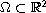be a bounded domain with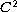boundary. In this paper, we are interested in the problem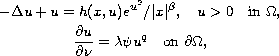where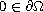,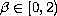,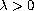,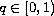and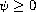is a H\"older continuous function on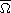. Here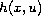is a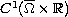having superlinear growth at infinity. Using variational methods we show that there exists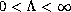such that above problem admits at least two solutions in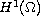if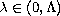, no solution if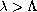and at least one solution when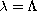.

Submitted August 26, 2008. Published March 24, 2009.
Math Subject Classifications: 34B15, 35J60
Key Words: Multiplicity; nonlinear Neumann boundary condition; Laplace equation.

Show me the PDF file (280 KB), TEX file, and other files for this article.

 Bhatia Sumit Kaur Department of Mathematics Indian Institute of Technology Delhi Hauz Khaz New Delhi-16, India email: sumit2212@gmail.com Konijeti Sreenadh Department of Mathematics Indian Institute of Technology Delhi Hauz Khaz New Delhi-16, India email: sreenadh@gmail.com